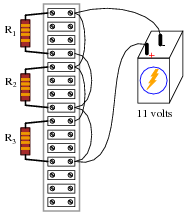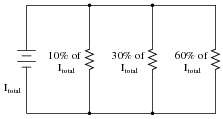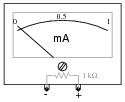# Current Divider Circuits

## DC Electric Circuits

• #### Question 1

 Don’t just sit there! Build something!!

Learning to mathematically analyze circuits requires much study and practice. Typically, students practice by working through lots of sample problems and checking their answers against those provided by the textbook or the instructor. While this is good, there is a much better way.

You will learn much more by actually building and analyzing real circuits, letting your test equipment provide the “answers” instead of a book or another person. For successful circuit-building exercises, follow these steps:

1. Carefully measure and record all component values prior to circuit construction.
2. Draw the schematic diagram for the circuit to be analyzed.
3. Carefully build this circuit on a breadboard or other convenient medium.
4. Check the accuracy of the circuit’s construction, following each wire to each connection point, and verifying these elements one-by-one on the diagram.
5. Mathematically analyze the circuit, solving for all values of voltage, current, etc.
6. Carefully measure those quantities, to verify the accuracy of your analysis.
7. If there are any substantial errors (greater than a few percent), carefully check your circuit’s construction against the diagram, then carefully re-calculate the values and re-measure.

Avoid very high and very low resistor values, to avoid measurement errors caused by meter “loading”. I recommend resistors between 1 kΩ and 100 kΩ, unless, of course, the purpose of the circuit is to illustrate the effects of meter loading!

One way you can save time and reduce the possibility of error is to begin with a very simple circuit and incrementally add components to increase its complexity after each analysis, rather than building a whole new circuit for each practice problem. Another time-saving technique is to re-use the same components in a variety of different circuit configurations. This way, you won’t have to measure any component’s value more than once.

• #### Question 2

We know that the voltage in a parallel circuit may be calculated with this formula:

 E = Itotal Rtotal

We also know that the current through any single resistor in a parallel circuit may be calculated with this formula:

 IR = E R

Combine these two formulae into one, in such a way that the E variable is eliminated, leaving only IR expressed in terms of Itotal, Rtotal, and R.

• #### Question 3

What will happen to the current through R1 and R2 if resistor R3 fails open?• #### Question 4

Determine the amount of current conducted by each resistor in this circuit, if each resistor has a color code of Org, Org, Red, Gld (assume perfectly precise resistance values - 0% error):Voltage across each resistor
Power dissipated by each resistor
Ratio of each resistor’s current to battery current (IR)/(Ibat)
Ratio of total circuit resistance to each resistor’s resistance (Rtotal)/R
• #### Question 5

Calculate the necessary resistor values to produce the following percentage splits in current:Hint: one resistor carries three times the current of the other.

• #### Question 6

Calculate one possible set of resistor values that would produce the following percentage splits in current:• #### Question 7

Calculate the percentage of total current for each resistor in this parallel circuit:• #### Question 8

Calculate the proper value of resistance R2 needs to be in order to draw 40% of the total current in this circuit:• #### Question 9

A student is trying to use the “current divider formula” to calculate current through the second light bulb in a three-lamp lighting circuit (typical for an American household):The student uses Joule’s Law to calculate the resistance of each lamp (240 Ω), and uses the parallel resistance formula to calculate the circuit’s total resistance (80 Ω). With the latter figure, the student also calculates the circuit’s total (source) current: 1.5 A.

Plugging this into the current divider formula, the current through any one lamp turns out to be:

 I = Itotal ( Rtotal R ) = 1.5 A ( 80 Ω 240 Ω ) = 0.5 A

This value of 0.5 amps per light bulb correlates with the value obtained from Joule’s Law directly for each lamp: 0.5 amps from the given values of 120 volts and 60 watts.

The trouble is, something doesn’t add up when the student re-calculates for a scenario where one of the switches is open:With only two light bulbs in operation, the student knows the total resistance must be different than before: 120 Ω instead of 80 Ω. However, when the student plugs these figures into the current divider formula, the result seems to conflict with what Joule’s Law predicts for each lamp’s current draw:

 I = Itotal ( Rtotal R ) = 1.5 A ( 120 Ω 240 Ω ) = 0.75 A

At 0.75 amps per light bulb, the wattage is no longer 60 W. According to Joule’s Law, it will now be 90 watts (120 volts at 0.75 amps). What is wrong here? Where did the student make a mistake?

• #### Question 10

Suppose an ammeter has a range of 0 to 1 milliamp, and an internal resistance of 1000 Ω:Show how a single resistor could be connected to this ammeter to extend its range to 0 to 10 amps. Calculate the resistance of this “range” resistor, as well as its necessary power dissipation rating.